﻿ 基于视觉信息融合技术的水面船只检测技术开发
 舰船科学技术2022, Vol. 44Issue (19): 134-137    DOI: 10.3404/j.issn.1672-7649.2022.19.026PDF

Development of surface ship detection technology based on visual information fusion technology
BAO Lin
Dalian Ocean University, Dalian 116023, China
Abstract: As a new kind of ship, unmanned ship has very important value in both military and commercial fields. The research direction of this paper is the development of ship target detection technology for surface unmanned ships. The principles of millimeter wave radar target detection technology and computer vision target recognition technology are introduced respectively. Based on the two target detection methods, a surface ship target detection technology based on information fusion is developed, which is described in detail from the aspects of time registration and space registration.
Key words: unmanned ship     target detection     information fusion technology     radar     computer vision
0 引　言

1）解析水面无人船的激光雷达目标探测技术，介绍激光雷达系统的基本原理，对激光雷达的信号进行数学建模；

2）研究水面无人船基于计算机视觉技术的目标探测技术，对计算机视觉的图像分割、图像滤波、特征提取等内容重点进行介绍；

3）提出一种基于视觉信息融合技术的水面船只目标检测技术，重点介绍激光雷达探测信号和计算机视觉信号的融合方法，从时间序列和空间配准等方面进行详细研究。

1 无人船的激光雷达目标检测技术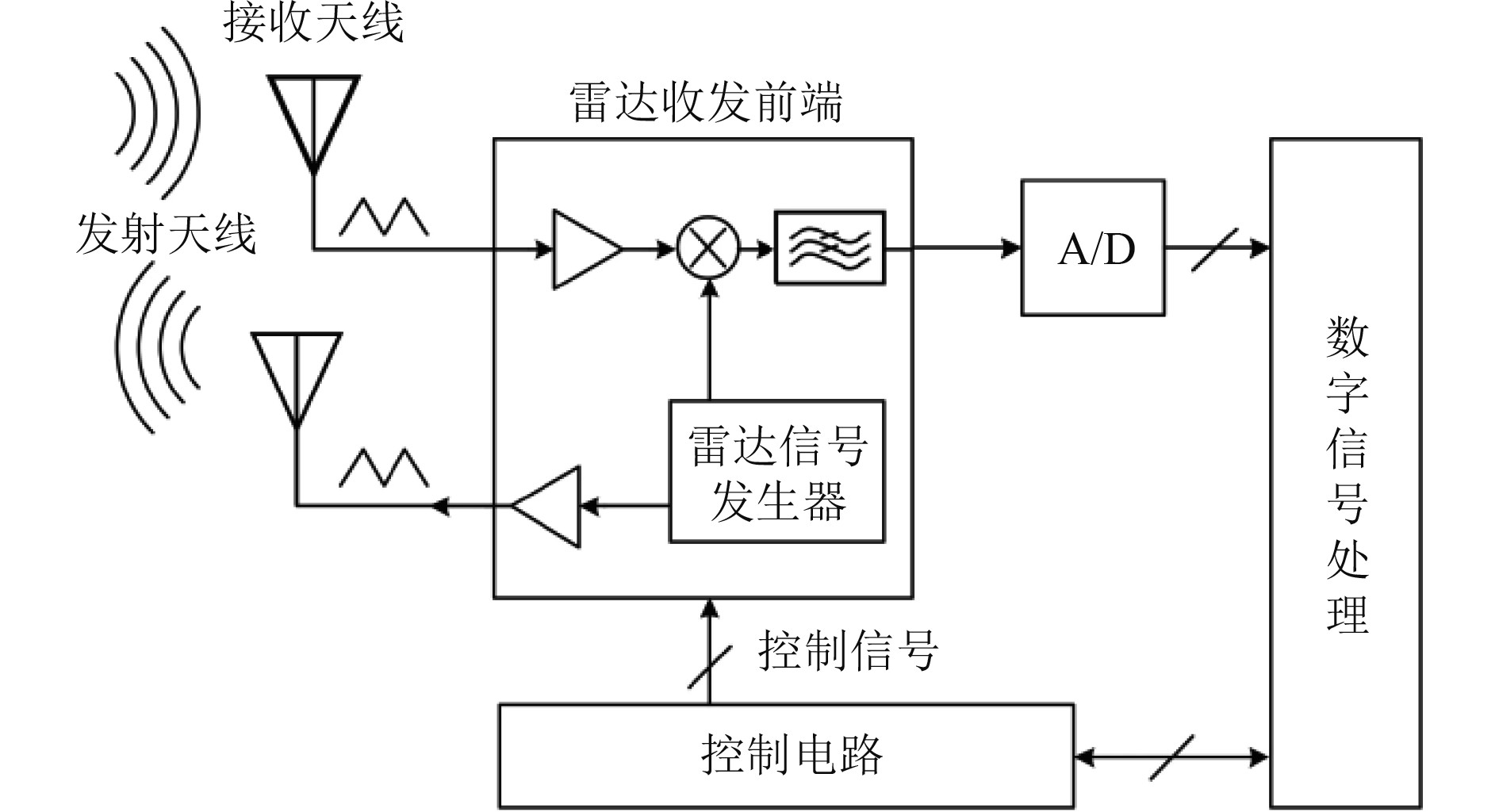图 1 毫米波雷达传感器的工作原理图 Fig. 1 Working principle diagram of millimeter wave radar sensor

 $f\left( t \right) = rect{\left( {\frac{t}{T}} \right)^{j\sqrt 2 \text{π} \left( {{f_0} + \frac{k}{2}{t^3}} \right)}} \text{。}$

 $rect\left( {\frac{t}{T}} \right) = \left\{ {\begin{array}{*{20}{c}} {0，\;\;\;\;\;\;\left| {\dfrac{t}{T}} \right| \leqslant 1}，\\ {1，\;\;\;\;\;\;\;\;{\rm{other}}} 。\end{array}} \right.$

 $\left\{ {\begin{array}{*{20}{l}} {{L_s}=\dfrac{1}{2}{R_0}\cos {\theta _\alpha }\cos {\theta _r} - {R_0}\sin {\theta _\alpha }} ，\\ {{\rm{W}}={R_0}\cos {\theta _\alpha } - {R_0}\sin {\theta _\alpha }} 。\end{array}} \right.$

 $f\left( t \right) = a\left( t \right)\cos \left[ {\sqrt 2 \text{π}i {f_0}t + \varphi \left( t \right)} \right]rect{\left( {\frac{t}{T}} \right)^{j\sqrt 2 \text{π} \left( \begin{subarray}{l} 0 \\ {h_0} + \frac{k}{2}{t^3} \end{subarray} \right)}} \text{。}$

2 无人船的计算机视觉目标探测技术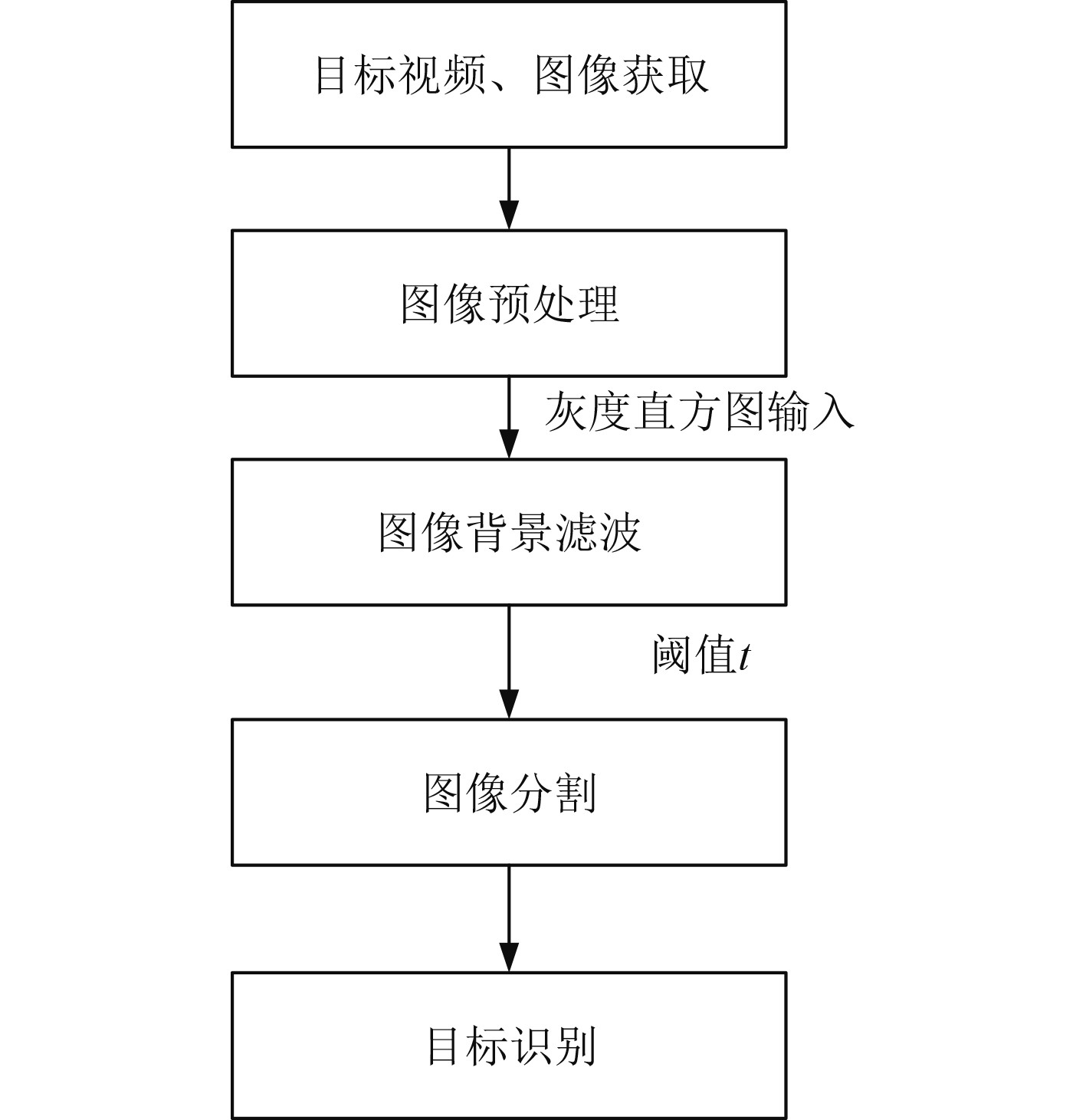图 2 无人舰船的计算机视觉目标探测流程 Fig. 2 Computer vision target detection process of unmanned ship

1）目标视频和图像获取

2）图像的预处理

 $\left[ {\begin{array}{*{20}{c}} Y \\ U \\ V \end{array}} \right] = \left[ {\begin{array}{*{20}{c}} {0.257}&{0.504}&{0.098} \\ {0.439}&{ - 0.368}&{ - 0.071} \\ { - 0.148}&{ - 0.291}&{0.439} \end{array}} \right]\left[ {\begin{array}{*{20}{l}} R \\ G \\ B \end{array}} \right] + \left[ {\begin{array}{*{20}{c}} {16} \\ {128} \\ {128} \end{array}} \right] \text{。}$

 $g(i,j) = \exp \left[ { - \frac{{\left( {{i^2} + {j^2}} \right)}}{{2{\sigma ^2}}}} \right] \text{。}$

3）图像背景滤波

 ${f_r}(x,y,t) = \left\{ {\begin{array}{*{20}{l}} {{f_e}(x,y,t)}，&{\left| {{f_e}(x,y,t) - {f_b}(x,y,t)} \right| \geqslant T}，\\ 0，&{\left| {{f_e}(x,y,t) - {f_b}(x,y,t)} \right| < T} 。\end{array}} \right.$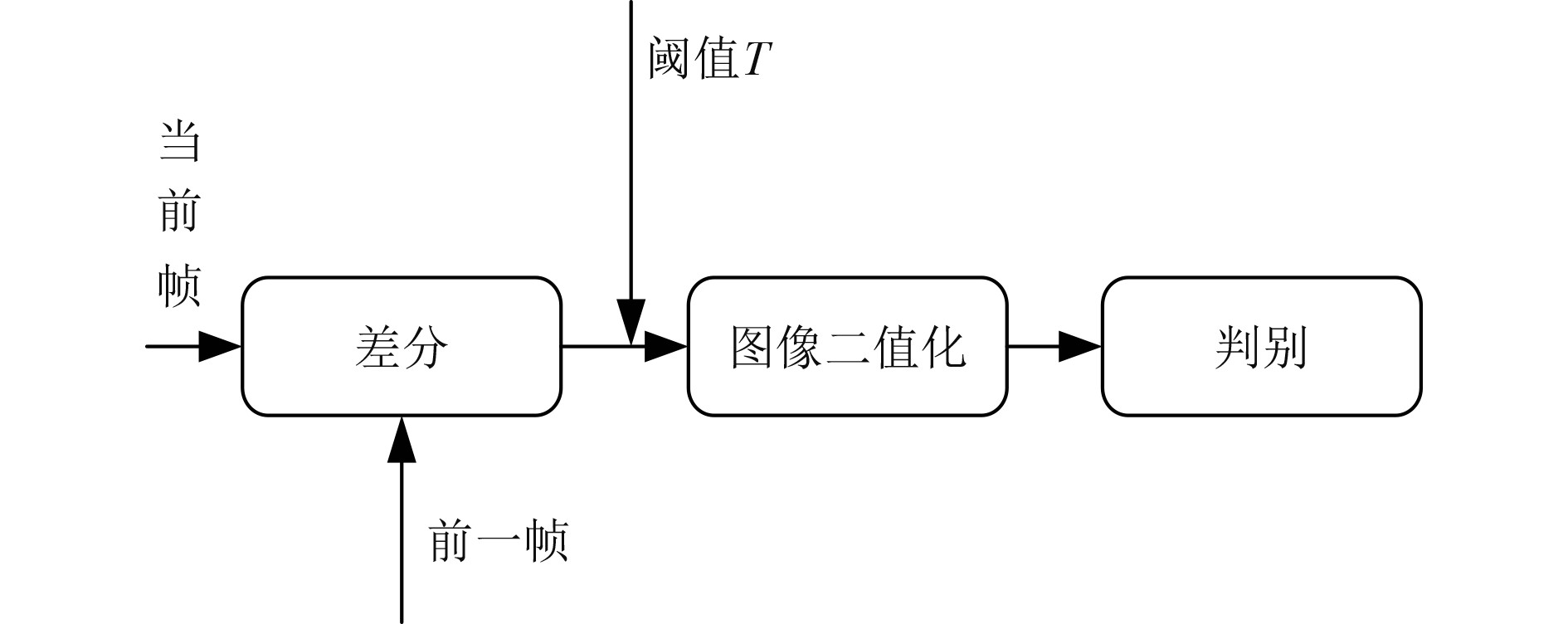图 3 图像背景滤波算法的原理 Fig. 3 Principle of image background filtering algorithm

4）图像分割和目标识别

3 基于视觉信息融合技术的无人船水面船只检测技术 3.1 雷达与计算机视觉探测技术的信息融合算法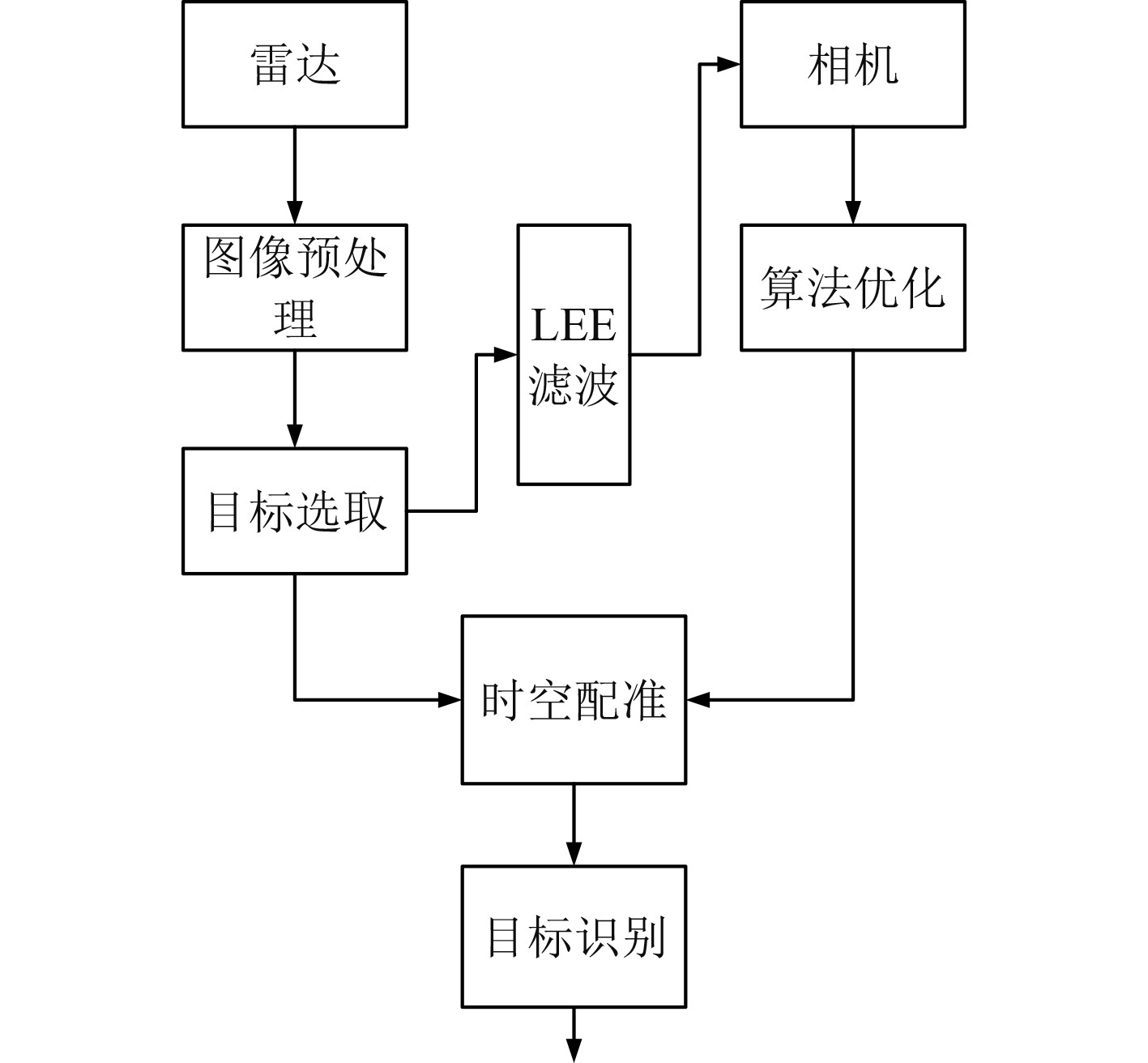图 4 雷达与计算机视觉探测的信息融合算法 Fig. 4 Information fusion algorithm of radar and computer vision detection

 $R\left( t \right) = \frac{{w\left( t \right) + 1}}{{I\left( t \right)\left( {1 - w\left( t \right)} \right)}} \text{。}$

 $\int\limits_R {\left| {\frac{{{\sigma _0}}}{{{\sigma _i}}}} \right|^2}{\rm{d}}t \leqslant \infty \text{。}$

LEE滤波器的小波变换能够提高噪声滤波的带宽，如下式：

 $F\left( {s,t} \right) = \left\{ {f\left( t \right),R\left( t \right)} \right\} = \frac{1}{{\sqrt s }}\int\limits_{}^{} {f\left( t \right)} R\left( {\frac{{t - \alpha }}{s}} \right){\rm{d}}t 。$

 $R\left( {s,t} \right) = \frac{1}{{{\alpha ^2}}}\int\nolimits_{ - \infty }^\infty {w\left( t \right)F\left( {s,t} \right)} R\left( {\frac{{t - \alpha }}{s}} \right){\rm{d}}t \text{。}$

3.2 无人船水面船只目标检测的时空对准

1）时间配准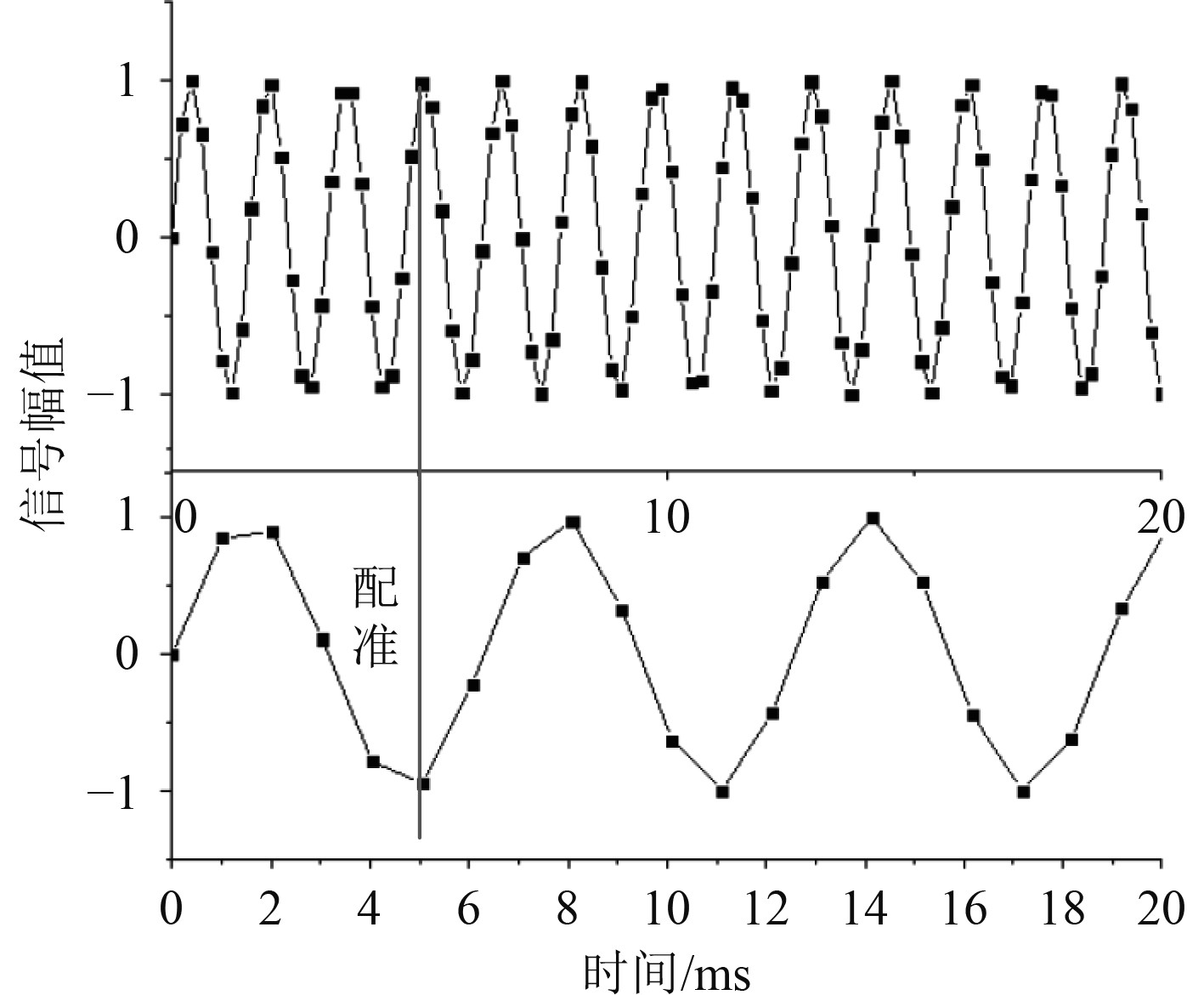图 5 水面船只探测的时间配准示意图 Fig. 5 Schematic diagram of time registration for surface ship detection

2）空间配准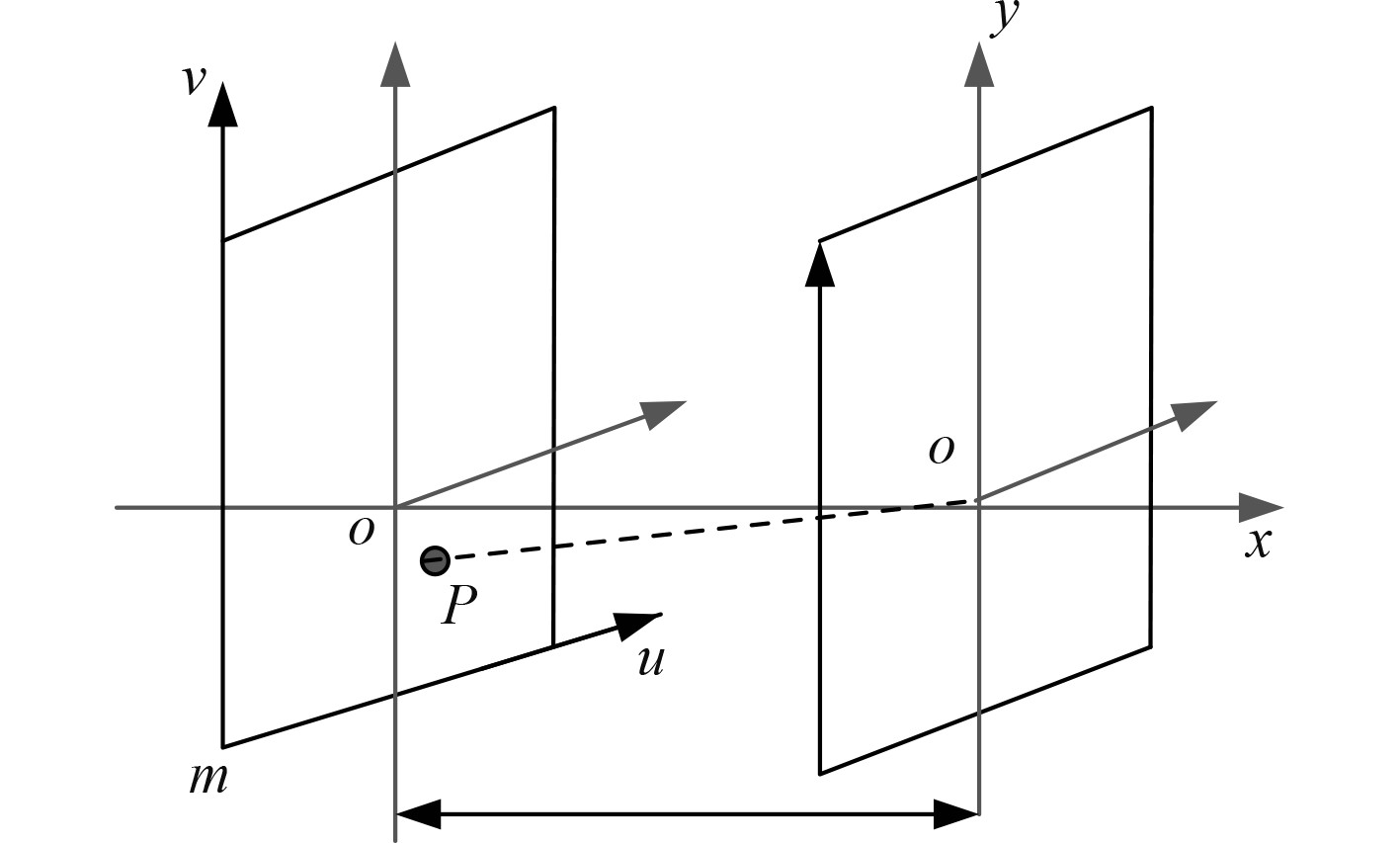图 6 空间配准坐标系 Fig. 6 Spatial registration coordinate system

 $\left[ {\begin{array}{*{20}{c}} u \\ v \\ l \end{array}} \right] = M\left[ {\begin{array}{*{20}{c}} {{X_w}} \\ {{Y_w} - m} \\ {{Z_w}} \\ 1 \end{array}} \right]\dfrac{1}{{{Z_w}}} = M\left[ {\begin{array}{*{20}{c}} {\dfrac{{R \times \sin \alpha }}{{{\text{n}} + R \times \cos \alpha }}} \\ {\dfrac{{ - h - m}}{{{\text{n}} + R \times \cos \alpha }}} \\ l \end{array}} \right] \text{。}$

4 结　语

  王萍. 基于数据特征的在线健康社区信息融合模式研究[J]. 现代情报, 2022, 42(8): 28-36+167. WANG Ping. Research on Information Fusion Mode of Online Healthy Community Based on Data Characteristics[J]. Modern Intelligence, 2022, 42(8): 28-36+167. DOI:10.3969/j.issn.1008-0821.2022.08.004  赵海萍. 无线传感器网络中的可靠信息融合模型研究[J]. 微型电脑应用, 2022, 38(7): 121-123+153. ZHAO Hai-ping. Research on Reliable Information Fusion Model in Wireless Sensor Networks[J]. Microcomputer Application, 2022, 38(7): 121-123+153. DOI:10.3969/j.issn.1007-757X.2022.07.034  张付东. 基于人工智能与多传感器信息融合的电缆火灾预警算法[J]. 电子设计工程, 2022, 30(6): 86–90. ZHANG Fu-dong. Cable fire early warning algorithm based on artificial intelligence and multi-sensor information fusion [J]. Electronic Design Engineering, 2022, 30(6): 86–90.